# HFTA-15.0 Thermistor Networks and Genetics

### Abstract

This application note discusses applications of thermistors for temperature compensation. It also gives a brief description of the genetic algorithm, which can quickly identify optimal circuit conditions. An example of the algorithm is used to illustrate how easy it is to determine the best resistor and NTC thermistor values to generate a particular voltage vs. temperature curve.

### Introduction

Question: Given the circuit topology in Figure 1, find the resistor and NTC thermistor values that generate the Voltage vs. Temperature curve shown in Figure 2. (Note: Only standard values may be used.)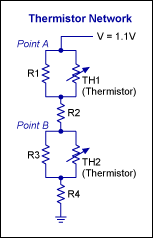Figure 1. Thermistor network.Figure 2. Voltage vs. temperature curve.

While this question may not appear in a textbook, it is a common practical problem that is encountered when trying to apply temperature compensation to an active device using a thermistor network. Some of us may be able to look at the circuit in Figure 1 and determine the solution by using a scratch pad and numerous equations. The rest of us would probably just enter the values into a simulator or spreadsheet and slowly try to adjust the values until we were able to match the curves as best as possible.

Finding the optimal solution to this circuit using either of the methods above can prove challenging, given the number of variables and the temperature dependence involved in its solution. Being constrained to only standard values for practical solutions increases the difficulty. While solving this circuit for one set of conditions may be acceptable, it can be tedious to solve for multiple conditions, networks, or desired responses. As the networks become more complicated (to provide a better temperature response), finding an optimal solution can often be too difficult or too time-consuming to solve symbolically or empirically. In contrast, a numerical method, such as the Genetic Algorithm, can solve these problems quickly and easily.

The words genetics and circuits rarely find themselves in the same context but, as will be shown, the genetic process can be applied to solve component values in circuits such as that shown in Figure 1. This article discusses applications of thermistors for temperature compensation and gives a brief description of the Genetic Algorithm. An example of the algorithm being used to quickly solve the basic question above will then be illustrated.

### Applications of Thermistor Networks

Thermistor networks are commonly used to apply temperature compensation to a wide variety of circuit applications. For example, thermistor networks are used to adjust power-supply circuits, PWM outputs, etc., as well as provide temperature-compensated bias currents to transistors, amplifier circuits, and laser diodes. Given the many applications for thermistor networks, the required compensation (network values) will vary greatly from application to application and from device to device.

Figure 2 is an example of the compensation needed for a laser diode used in a fiber-optic communication link application. To maintain the quality of the communication link, the bias current to the laser diode must be adjusted as temperature changes. The temperature-compensated bias current can be applied to the laser by using the circuit shown in Figure 3, but the optimal values must be determined to match the desired voltage vs. temperature (Figure 2). Using the Genetic Algorithm, a near-ideal solution can be found in a matter of seconds.Figure 3. Thermistor network with the MAX3643 burst-mode laser driver.

### The Genetic Algorithm

The Genetic Algorithm is an optimization algorithm that mimics the genetic process to find optimal solutions to multivariable problems. Although the Genetic Algorithm was first used in the 1950s,¹ it has only been widely used in the last 15 to 20 years due to advances in computer technology and processor speed.

In its simplest form, the algorithm starts by creating a random population or a population of predetermined starting points. Each population element (individual) in a population (Figure 4) is composed of an array of variables that defines its construction (its genetic code). Once each population element is initialized, its performance is measured using a set of criteria and then compared against all other elements. The population elements are then sorted according to their performance. The elements with a poor ranking are eliminated (natural selection), and a new generation of elements is created by mixing the variables (genetic code) of the best population elements from the previous generation (Figure 5).Figure 4. Illustration of the population with K population elements, each composed of N variables.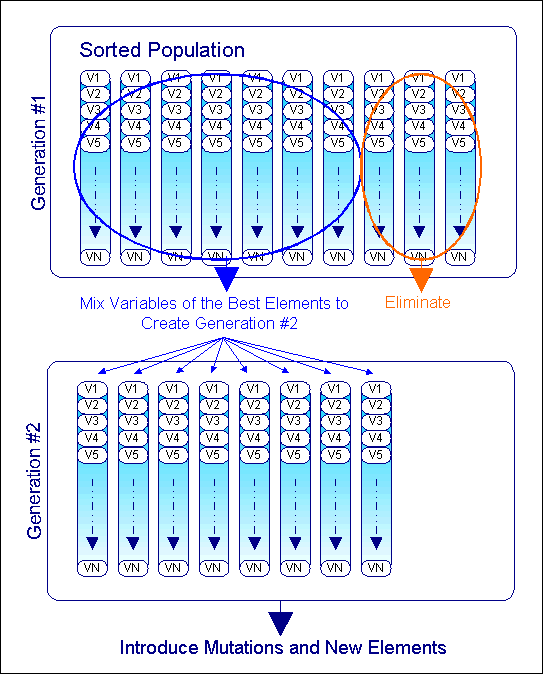Figure 5. Illustration of the process of mixing the variables (i.e., the genetic code) from one generation to the next.

If the process were allowed to loop after completing the steps to this point, the population would quickly saturate to a common, identical genetic code. A minimum will be found, but it may not be the global minimum. To keep the population from saturating or converging on a local minimum, mutations (random changes) to a population element's variables and new random elements should be introduced into the population (Figure 6).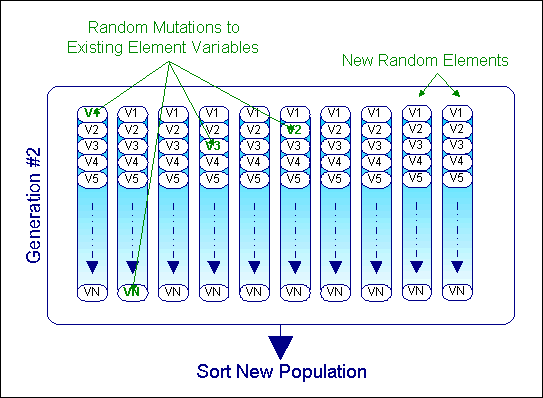Figure 6. Illustration of the process of introducing random elements into a generation's population.

The frequency and amount of randomness that should be introduced will vary from one application to another. If too much randomness is introduced, the algorithm will not have time to converge on an ideal solution. If too little is introduced, finding the global minimum could take an extremely long time. The amount of randomness introduced should, therefore, be tuned by trial and error or set dynamically with more randomness introduced as the population saturation increases.

There are other optimization algorithms that can be faster than the Genetic Algorithm; however, they are often more complicated to program for multivariable problems and they will often converge on a local minimum. The Genetic Algorithm overcomes these problems.

The Genetic Algorithm is based on a random process, so the time needed to converge on an absolute optimal solution will be random. The average convergence time will increase as the number of variables in each population element increases. Determining and calculating the performance criteria for a given application can also be difficult for some applications. Simply put, it is sometimes difficult to write equations that express what the optimal solution should look like. For simple passive circuits such as the thermistor circuit shown in Figure 1, the performance criteria can be well defined and easily written. Given the number of variables, the solution can also be found in a reasonable amount of time. The Genetic Algorithm is, therefore, well suited to solve these types of circuits.

### Example

Using a free visual basic compiler,², a simple routine was written to solve the thermistor network values (Figure 1) to match the desired response (Figure 2). The source code for this example and the executable file can be downloaded.

The algorithm is implemented in the software with the following basic steps:

1. Define the available resistor and thermistor values that can be used as possible variables in each population element. An array is declared for available resistors and another for available thermistors.
2. Generate a random population of elements. (Population should be composed of 100 elements for this example.) Each population element is an array that contains an entry for each component in the circuit (Figure 1).
3. Equate the desired voltage or resistance of the network over temperature and graph its response. The desired voltage/resistance is then imported into the code using a polynomial equation.
4. Calculate the mean square error of each population element.
5. Sort the population from lowest error to highest error and graph the best solution.
6. Eliminate the worst solution and randomly or methodically combine the remaining elements to create a second generation of population elements.
7. Introduce randomness by introducing new random population elements or by randomly changing one or more variables in one or more of the existing population elements.
8. Return to step 4 and repeat until the mean square error is sufficiently low and the user decides to stop the process.
At this point you may be asking yourself, "How does this solve the network values?" Just as with genetics and evolution, the solution "grows" from one state to another. The optimal solution is essentially the best genetic code (circuit element values) to the environment (desired circuit response). If you are still shaking your head, it is okay. Your worries will soon be resolved with the following example results.

The software's graphical user interface is shown in Figure 7. From this interface, you can select the thermistor network configuration and input the desired response using a polynomial expression. You can select to solve for total resistance or a voltage, the temperature range to optimize, and if 1% or 5% resistor values should be used. Pressing the "Run" button starts the algorithm. The mean square error, iteration count, and the component values for the current best solution are then updated as the program runs.Figure 7. Software makes the process of determining the thermistor values to match the desired response very simple.

A graph is also drawn showing the desired response (in green) as well as the current best solution (shown in red). The graphical output after the computer has run 30 iterations is shown in Figure 8. As seen in this figure, the response is nonideal. After 500 iterations, which take approximately 10 seconds, a very good response has been generated (Figure 9). Letting the program run while you take a quick break, reveals the results shown in Figure 10, which is almost an exact match.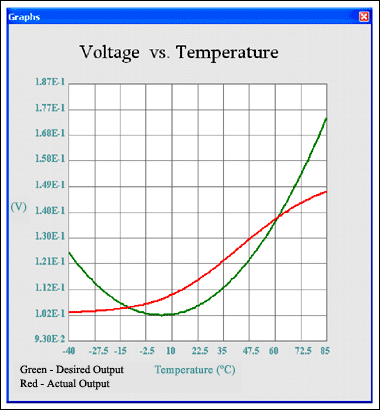Figure 8. Voltage vs. temperature curve shows the actual and desired output after 30 iterations.Figure 9. Voltage vs. temperature curve shows the actual and desired output after 500 iterations.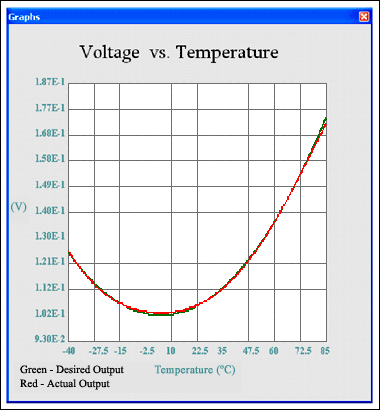Figure 10. Voltage vs. temperature curve shows how the actual and desired output now match.

Using Figure 1 as a reference, this solution is composed of the following component values:

• R1 = 8.2k, R2 = 3.6k, R3 = 2.2k, R4 = 1.1k
• TH1 = 47k Beta = 4700, TH2 = 40 Beta = 2750
The speed of convergence will vary from one test to another. It will converge much faster with a simpler network; it will take longer in general to converge with a more complicated network or if 1% resistor values are used because there are more variables to choose among.

Putting this all into perspective, a network composed of two thermistors and four resistors was closely matched to the desired temperature response in less than 30 seconds using only standard component values.

If you are still shaking your head trying to understand how genetics have been used to solve a circuit problem, then please download the program and try it out. You will be amazed at how quickly solutions to thermistor networks can be found using the Genetic Algorithm.

References

1. www.wikipedia.com, Genetic Algorithm.